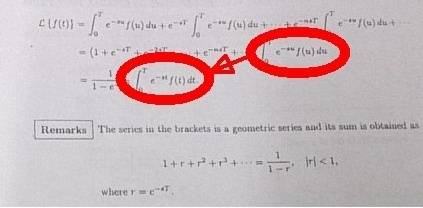# Laplace transform

for the right part of the notes, why the integral of (e^-su)f(u) from 0 to T will become integral of (e^-st)f(t) from 0 to T suddenly ? why not integral of (e^-s (t-nT) )f(t-nT) from 0 to T ? as we can see, u = t +nTd given/known data

## The Attempt at a Solution

#### Attachments

•DSC_0405.JPG
35.2 KB · Views: 326

Samy_A
Homework Helper
for the right part of the notes, why the integral of (e^-su)f(u) from 0 to T will become integral of (e^-st)f(t) from 0 to T suddenly ? why not integral of (e^-s (t-nT) )f(t-nT) from 0 to T ? as we can see, u = t +nTd given/known data

## The Attempt at a SolutionIf so, that's just renaming the (dummy) integration variable from u to t.

View attachment 95345
If so, that's just renaming the (dummy) integration variable from u to t.
yes , why thgere is no need to turn u into t -nT ? since it is given at the left part of the notes

Samy_A
Homework Helper
yes , why thgere is no need to turn u into t -nT ? since it is given at the left part of the notes
It's just a name. It doesn't matter whether the integration variable is called u or t (or something else).

Example: the two following integrals are equal
##\displaystyle \int_a^b e^x dx = \int_a^b e^y dy##

Last edited:
vela
Staff Emeritus